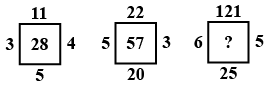### SSC JE Mechanical Engineering 25th May 2014 Shift-2 Question 28

Instructions

In the following questions find the missing number from the given responses.

Question 28

#Solution

(11 + 5) + 3 $$\times$$ 4 = 16 + 12 = 28
(22 + 20) + 5 $$\times$$ 3 = 42 + 15 = 57
(121 + 25) + 6 $$\times$$ 5 = 146 + 30 = 176
$$\therefore$$ The correct answer is option A.

• Free SSC Study Material - 18000 Questions
• 230+ SSC previous papers with solutions PDF